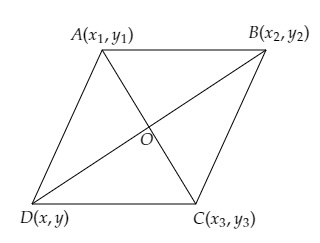# $ABCD$ is a parallelogram with vertices $A (x_1, y_1), B (x_2, y_2)$ and $C (x_3, y_3)$. Find the coordinates of the fourth vertex $D$ in terms of $x_1, x_2, x_3, y_1, y_2$ and $y_3$.

Given:

$ABCD$ is a parallelogram with vertices $A (x_1, y_1), B (x_2, y_2)$ and $C (x_3, y_3)$.

To do:

We have to find the fourth vertex $D$ in terms of $x_1, x_2, x_3, y_1, y_2$ and $y_3$.

Solution:

Let the fourth vertex be $D(x,y)$ and the diagonals $AC$ and $BD$ bisect each other at $O$.This implies,

$\mathrm{O}$ is the mid-point of $\mathrm{AC}$.

The coordinates of $\mathrm{O}$ are $(\frac{x_1+x_3}{2}, \frac{y_1+y_3}{2})$

$\mathrm{O}$ is the mid-point of $\mathrm{BD}$.

The coordinates of $\mathrm{O}$ are $(\frac{x_2+x}{2}, \frac{y_2+y}{2})$

Therefore,

$(\frac{x_1+x_3}{2}, \frac{y_1+y_3}{2})=(\frac{x_2+x}{2}, \frac{y_2+y}{2})$

On comparing, we get,

$\frac{x_1+x_3}{2}=\frac{x_2+x}{2}$

$\Rightarrow x_1+x_3=x_2+x$

$\Rightarrow x=x_1+x_3-x_2$

Similarly,

$\frac{y_1+y_3}{2}=\frac{y_2+y}{2}$

$\Rightarrow y_1+y_3=y_2+y$

$\Rightarrow y=y_1+y_3-y_2$

Therefore, the coordinates of the fourth vertex are $(x_1+x_3-x_2, y_1+y_3-y_2)$.

Updated on: 10-Oct-2022

54 Views